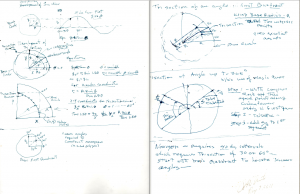﻿ Roll Grinding Measurement Solutions| » TRISECTING AN ANGLE

### TRISECTING AN ANGLETRISECTING AN ANGLE, USING ONLY AN UNMARKED STRAIGHT EDGE AND A COMPASS.

“IMPOSSIBLE”, SAY THE EXPERTS – “SIMPLE “, SAYS THIS TRISECTOR

(Mystery and Solution)

ABSTRACT: This thesis presents solutions to four drafting puzzles that date back to Ancient Greece and are universally considered to be insolvable. These are: Trisecting an Angle: Constructing a Nonagon: Squaring the Circle and Doubling the Cube. These seemingly simple tasks are problems because the draftsman can use only a compass and an unmarked straight edge as his drawing tools. Remarkably, the graphical solutions illustrated in this paper show that each puzzle has a single line solution which properly located on its basic puzzle diagram, proves to be the key to/or the direct solution to that geometric problem. For each graphical solution drawings are shown and where necessary, mathematical equations are presented to substantiate the accuracy of the solutions. Finally, the writer concludes that while the solutions submitted are an achievement, the study and methodological approaches taken to solve these ancient problems are instructive and have as much merit as the solutions.